# Mechanics LecturePage 4

#### WATCH ALL SLIDES

Force:

Start from Newton’s 2nd Law

Magnitude:

Direction: toward the center of the circle of motion

Slide 25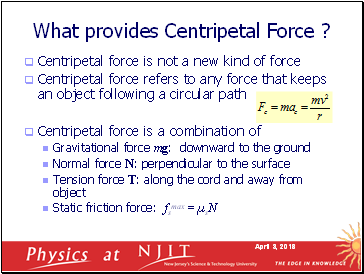## What provides Centripetal Force ?

Centripetal force is not a new kind of force

Centripetal force refers to any force that keeps an object following a circular path

Centripetal force is a combination of

Gravitational force mg: downward to the ground

Normal force N: perpendicular to the surface

Tension force T: along the cord and away from object

Static friction force: fsmax = µsN

Slide 26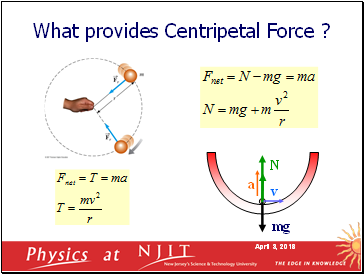a

What provides Centripetal Force ?

mg

N

Slide 27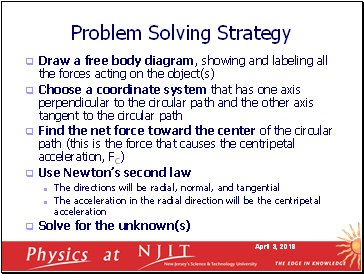## Problem Solving Strategy

Draw a free body diagram, showing and labeling all the forces acting on the object(s)

Choose a coordinate system that has one axis perpendicular to the circular path and the other axis tangent to the circular path

Find the net force toward the center of the circular path (this is the force that causes the centripetal acceleration, FC)

Use Newton’s second law

The directions will be radial, normal, and tangential

The acceleration in the radial direction will be the centripetal acceleration

Solve for the unknown(s)

Slide 28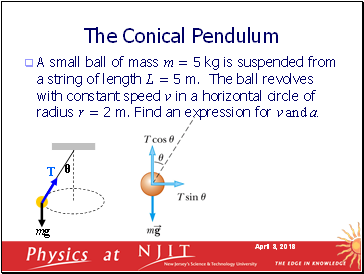## The Conical Pendulum

A small ball of mass m = 5 kg is suspended from a string of length L = 5 m. The ball revolves with constant speed v in a horizontal circle of radius r = 2 m. Find an expression for v and a.

mg

T

θ

Slide 29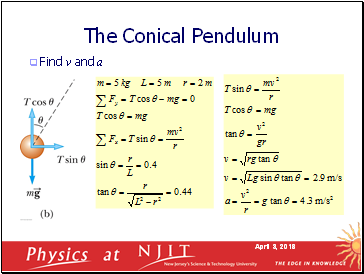The Conical Pendulum

Find v and a

Slide 30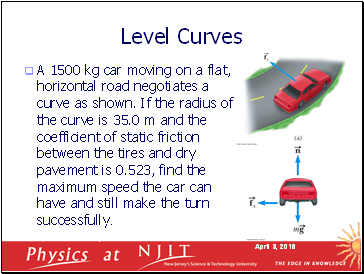## Level Curves

A 1500 kg car moving on a flat, horizontal road negotiates a curve as shown. If the radius of the curve is 35.0 m and the coefficient of static friction between the tires and dry pavement is 0.523, find the maximum speed the car can have and still make the turn successfully.

Slide 31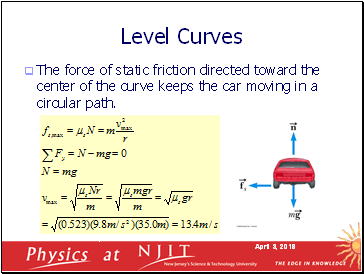Level Curves

The force of static friction directed toward the center of the curve keeps the car moving in a circular path.

Slide 32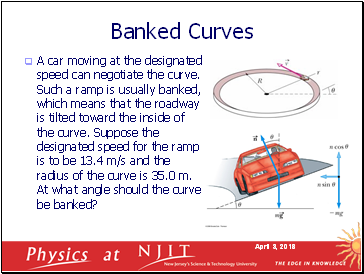## Banked Curves

A car moving at the designated speed can negotiate the curve. Such a ramp is usually banked, which means that the roadway is tilted toward the inside of the curve. Suppose the designated speed for the ramp is to be 13.4 m/s and the radius of the curve is 35.0 m. At what angle should the curve be banked?

Go to page:
1  2  3  4  5